CBSE Class 10 Sample Paper for 2020 Boards - Maths Basic

Class 10
Solutions of Sample Papers for Class 10 Boards

## A (3, 4), B (6, 7), C (9, 4), D (6, 1) respectively. Then a new student Anjali joins the class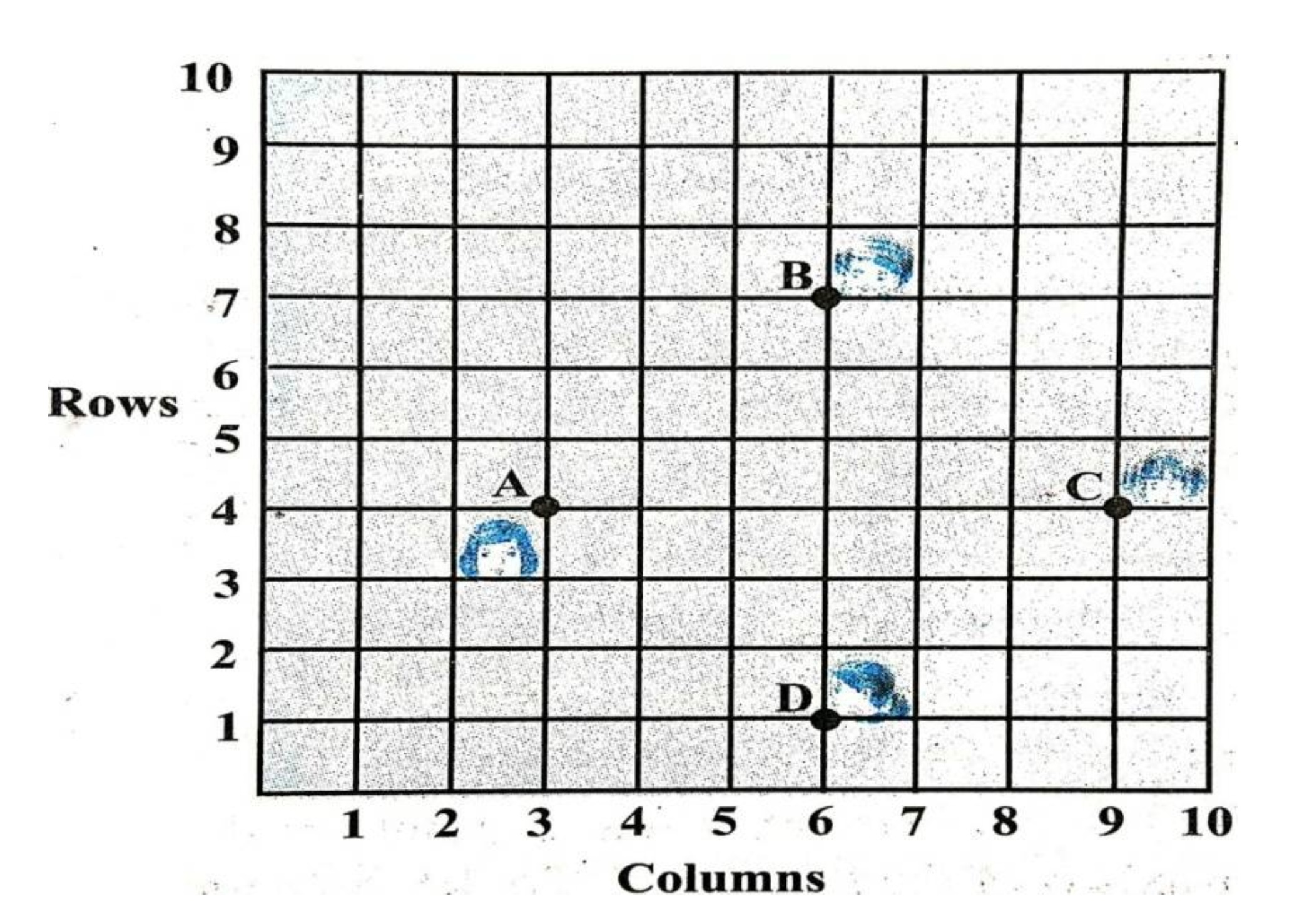## (iii) Which two students are equidistant from Gita.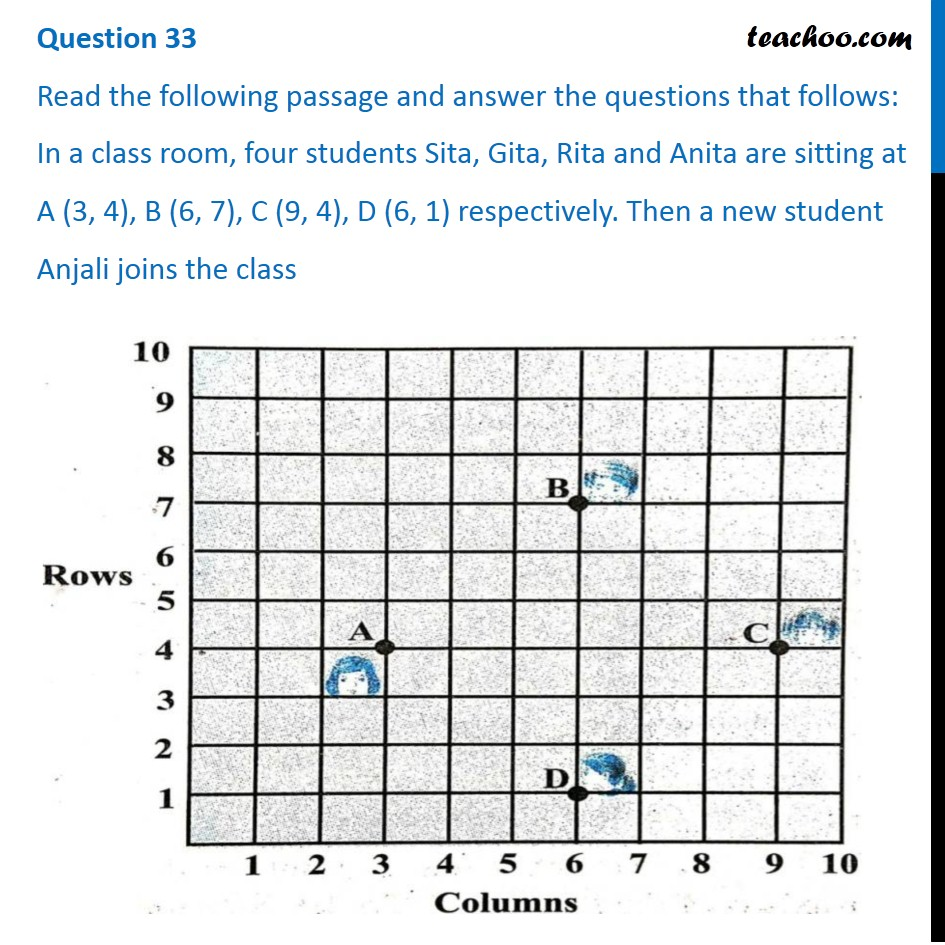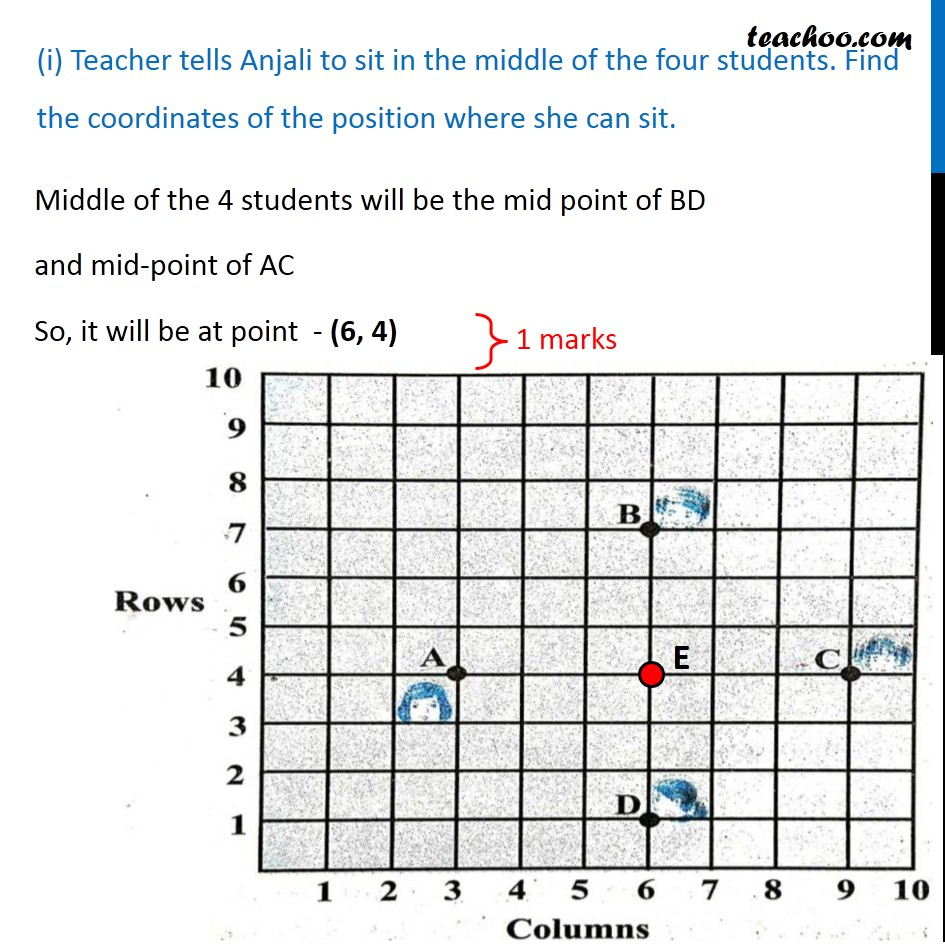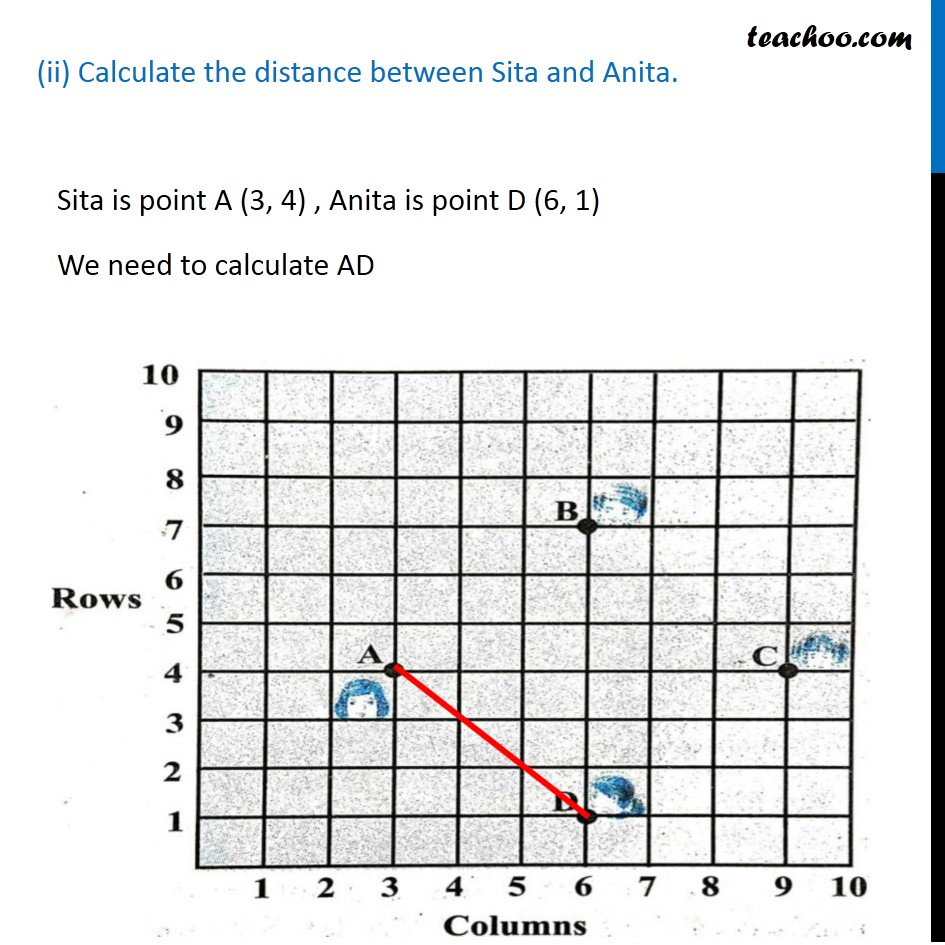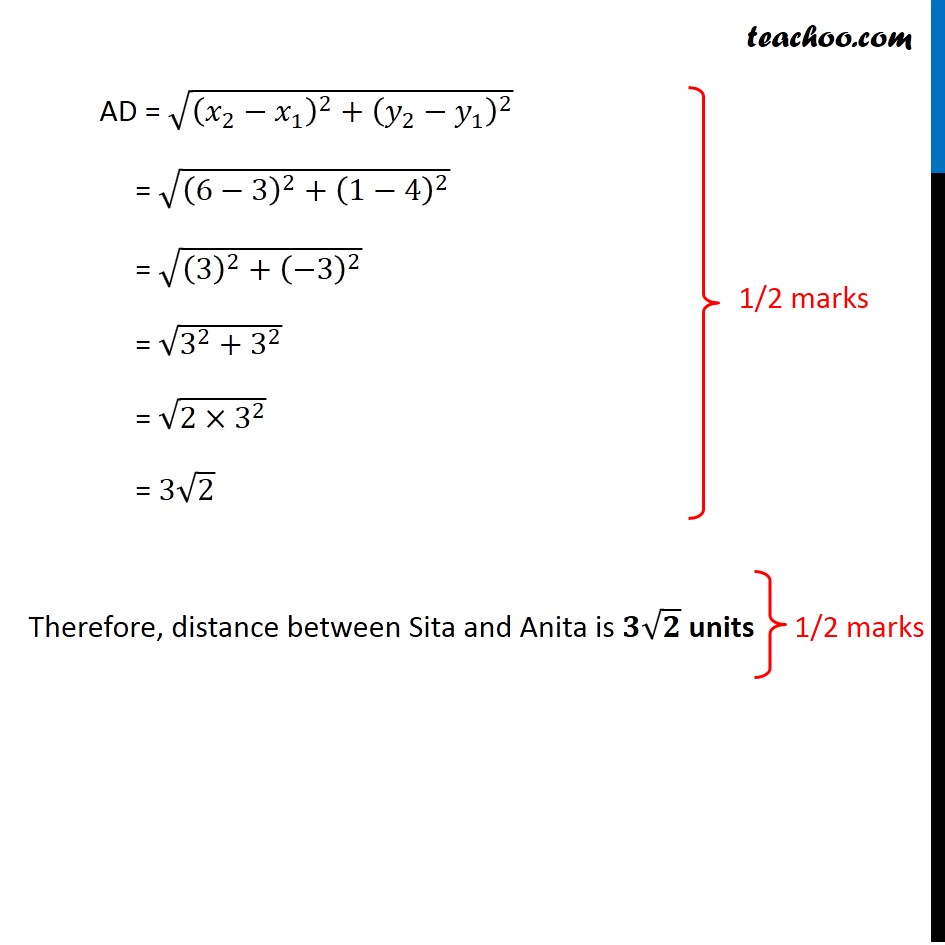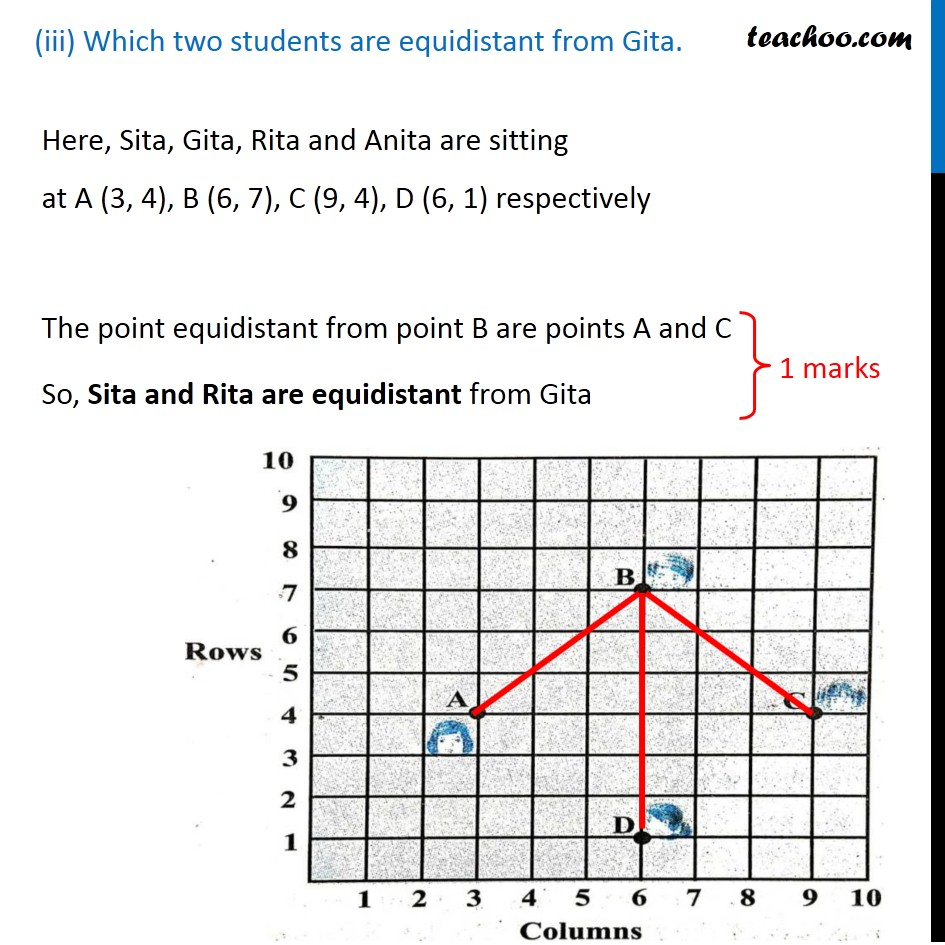Learn in your speed, with individual attention - Teachoo Maths 1-on-1 Class

### Transcript

Question 33 Read the following passage and answer the questions that follows: In a class room, four students Sita, Gita, Rita and Anita are sitting at A (3, 4), B (6, 7), C (9, 4), D (6, 1) respectively. Then a new student Anjali joins the class (i) Teacher tells Anjali to sit in the middle of the four students. Find the coordinates of the position where she can sit. Middle of the 4 students will be the mid point of BD and mid-point of AC So, it will be at point - (6, 4) (ii) Calculate the distance between Sita and Anita. Sita is point A (3, 4) , Anita is point D (6, 1) We need to calculate AD = √((𝑥_2−𝑥_1 )^2+(𝑦_2−𝑦_1 )^2 ) = √((0−3)^2+(0−(−4))^2 ) = √((−3)^2+(−4)^2 ) = √(3^2+4^2 ) = √(9+16) = √25 AD = √((𝑥_2−𝑥_1 )^2+(𝑦_2−𝑦_1 )^2 ) = √((6−3)^2+(1−4)^2 ) = √((3)^2+(−3)^2 ) = √(3^2+3^2 ) = √(2×3^2 ) = 3√2 Therefore, distance between Sita and Anita is 𝟑√𝟐 units (iii) Which two students are equidistant from Gita. Here, Sita, Gita, Rita and Anita are sitting at A (3, 4), B (6, 7), C (9, 4), D (6, 1) respectively The point equidistant from point B are points A and C So, Sita and Rita are equidistant from Gita